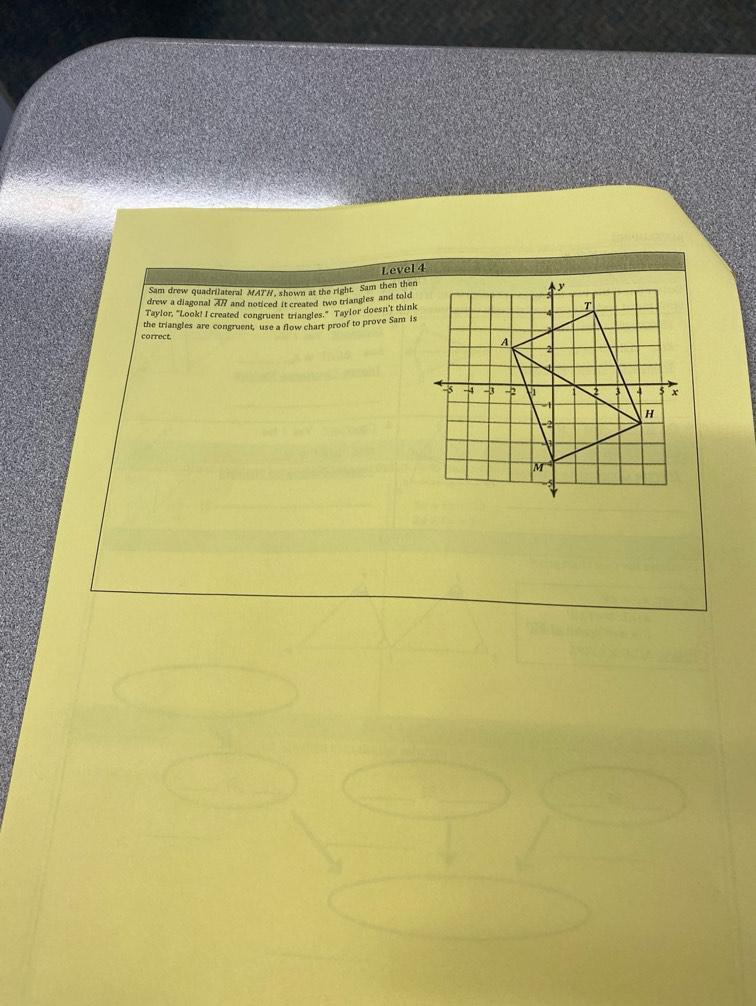Question:

# Level 4 Sum drew quadrilateral MATW, shown at the right. Sam then then drew a diagonal A and noticed it created two triangles anLevel 4 Sum drew quadrilateral MATW, shown at the right. Sam then then drew a diagonal A and noticed it created two triangles and trick Taylor. "Look! I created congruent triangles . Taylor doesn't think the triangles are congruent use a flow chart proof to prove Sam is correct H